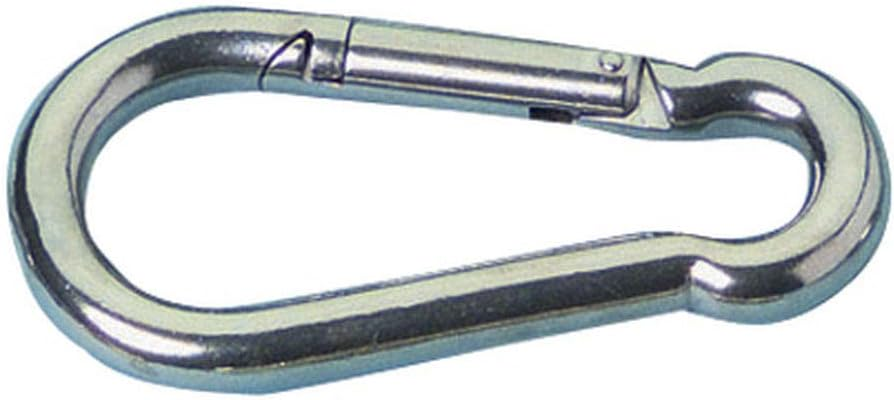\$48

Product description

Not to be used for overhead lifting.

Specifications:
4x40mm (1-1/2” x 5/32”) : A=4mm, B=20mm, C=40mm, D=7mm, F=6mm, WLL=100 lbs
5x50mm (2” x 3/16”) : A=5mm, B=25mm, C=50mm, D=8mm, F=7mm, WLL=160 lbs
6x60mm (2-1/2” x 1/4”) : A=6mm, B=30mm, C=60mm, D=9mm, F=8mm, WLL=260 lbs
7x70mm (2-3/4” x 9/32”) : A=7mm, B=35mm, C=70mm, D=10mm, F=10mm, WLL=335 lbs
8x80mm (3” x 5/16”) : A=8mm, B=40mm, C=80mm, D=12mm, F=11mm, WLL=400 lbs
9x90mm (3-3/4” x 11/32”) : A=9mm, B=45mm, C=90mm, D=12mm, F=11mm, WLL=500 lbs
10x100mm (4” x 3/8”) : A=10mm, B=50mm, C=100mm, D=15mm, F=12mm, WLL=650 lbs
11x120mm (4-3/4” x 7/16”) : A=11mm, B=57mm, C=120mm, D=18mm, F=19mm, WLL=700 lbs
12x140mm (5-1/2” x 1/2”) : A=12mm, B=67mm, C=140mm, D=20mm, F=20mm, WLL=800 lbs
13x160mm (6-1/4” x 1/2”) : A=13mm, B=74mm, C=160mm, D=22mm, F=29mm, WLL=1300 lbs
14x180mm (7” x 9/16”) : A=14mm, B=86mm, C=180mm, D=22mm, F=38mm, WLL=1600 lbs